Next: About this document ... Up: lab_template Previous: lab_template

Subsections

# Limits

## Introduction

Limits of many functions and expressions can be computed in Maple with the limit command.
> limit(sin(x)/x,x=0);
> g:=x->(3*x^2+4)/(x^2+7*x+12);
> limit(g(x),x=-2);

Maple can also do one-sided limits. Use Maple's online help to find out how to handle such limits. The following is the definition of the limit.

## Definition

To say thetmeans that for each
(no matter how small), there is a corresponding
such thatprovided that. This definition may seem complicated, but it has a nice graphical interpretation. Plot f with the y-range set to
and the x-range set to
. Try to choose
small enough so that the graph of f stays between the top and the bottom of the plot. If you can find such ano matter how small the
, then the limit exists and is equal to L. Consider this example. Let
and
Then any value forsmaller than about 0.049 will work. To see why this is so look at the plots genersted by the following commands.
> f:=x->x^2;
> limit(f(x),x=2);
> plot([4-0.2,4+0.2,f(x)],x=2-0.1..2+0.1,y=4-0.2..4+0.2);
> plot([4-0.2,4+0.2,f(x)],x=2-0.048..2+0.048,y=4-0.2..4+0.2);

In the first two plot commands, the value ofis 0.1. This is too large since the graph intersects the lines
and. The 0.048 value forin the second plot command, however, is small enough since the graph of f goes out the sides of the plot. Make sure you understand what is done in this example since you will need to do similar work in some of the exercises.

## Exercises

In exercises 1 and 2, use the limit command to find
. Then experiment with some plots to find athat works for the given. Also, find athat doesn't work. These twovalues should be close to each other. Thus yourthat works should be near the largest value ofthat can be used. Although you will probably need to do many plots, submit only the two required plots on your report.
1.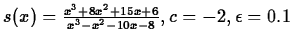2.
3. Consider the following limit statement
. If
, find and appropriate. Repeat your procedure with
.What conclusion do you draw from your work? Why?Next: About this document ... Up: lab_template Previous: lab_template
Jane E Bouchard
2004-10-28